﻿ Constitutional descriptors
 Constitutional descriptors

Constitutional descriptors are the most simple and commonly used descriptors, reflecting the chemical composition of a compound without any information about its molecular geometry or atom connectivity.

dProperties calculates some constitutional descriptors; many of them are well explained by their definition such as the molecular weight (MW), number of atoms (nAT), number of Hydrogen atoms (nH), number of Carbon atoms (nC), number of Nitrogen atoms (nN), number of Oxygen atoms (nO), number of halogen atoms (nX).

For the less trivial descriptors some more explanation is given below.

The number of rotatable bonds (RBN) is the number of bonds which allow free rotation around themselves. These are defined as any single bond, not in a ring, bound to a nonterminal heavy atom. Excluded from the count are amide C–N bonds because of their high rotational energy barrier (F. Veber et al., J.Med.Chem. 2002, 45, 2615-2623).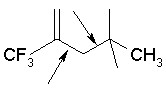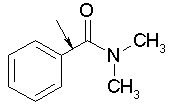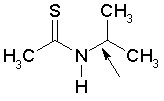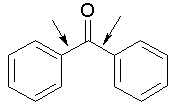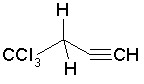RBN = 2 RBN = 1 RBN = 1 RBN = 2 RBN = 0

The number of rings (nCIC) counts the rings in a molecule. The number of rings (or independent cycles, i.e., the number of non-overlapping cycles) in a graph is commonly known as the cyclomatic number. The number of rings (nCIC) is calculated as the cardinality of the set of independent rings called the Smallest Set of Smallest Rings (SSSR).

The number of donor atoms for H-bonds (nHDon) is a measure of the hydrogen-bonding ability of a molecule expressed in terms of number of possible hydrogen-bond donors. Specifically, it is calculated by adding up the hydrogens bonded to any nitrogen and oxygen without negative charge in the molecule.

The number of acceptor atoms for H-bonds (nHAcc) is a measure of the hydrogen-bonding ability of a molecule expressed in terms of number of possible hydrogen-bond acceptors. Specifically, it is calculated by adding up any nitrogen, oxygen and fluorine, excluding N with positive formal charge, higher oxidation states and pyrrolyl form of nitrogen.